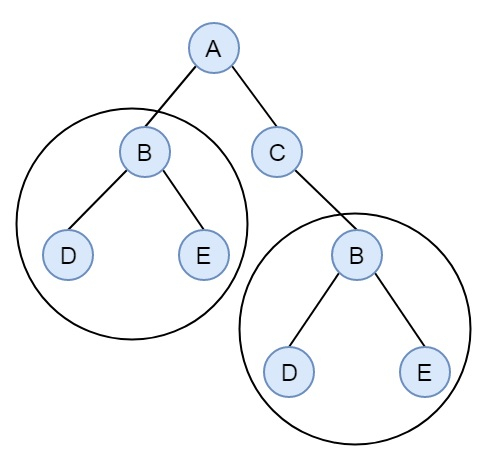# Find All Duplicate Subtrees in C++

Consider we have a binary tree. We have to find if there are some duplicate subtrees in the tree or not. Suppose we have a binary tree like below −There are two identical subtrees of size 2. In each subtree D, BD and BE both are also duplicate subtrees We can solve this problem by using tree serialization and hashing process. We will store the inorder traversal of subtrees in the hash table. We will insert opening and closing parenthesis for empty nodes.

## Example

Live Demo

#include <iostream>
#include <unordered_set>
#include <unordered_map>
#include <algorithm>
using namespace std;
const char MARKER = '\$';
struct Node {
public:
char data;
Node *left, *right;
};
Node* getNode(char key) {
Node* newNode = new Node;
newNode->data = key;
newNode->left = newNode->right = NULL;
return newNode;
}
unordered_set<string> subtrees;
string inorder(Node* node, unordered_map<string, int>& map) {
if (!node)
return "";
string str = "(";
str += inorder(node->left, map);
str += to_string(node->data);
str += inorder(node->right, map);
str += ")";
if (map[str] == 1)
cout << node->data << " ";
map[str]++;
return str;
}
void duplicateSubtreeFind(Node *root) {
unordered_map<string, int> map;
inorder(root, map);
}
int main() {
Node *root = getNode('A');
root->left = getNode('B');
root->right = getNode('C');
root->left->left = getNode('D');
root->left->right = getNode('E');
root->right->right = getNode('B');
root->right->right->right = getNode('E');
root->right->right->left= getNode('D');
duplicateSubtreeFind(root);
}

## Output

D E B